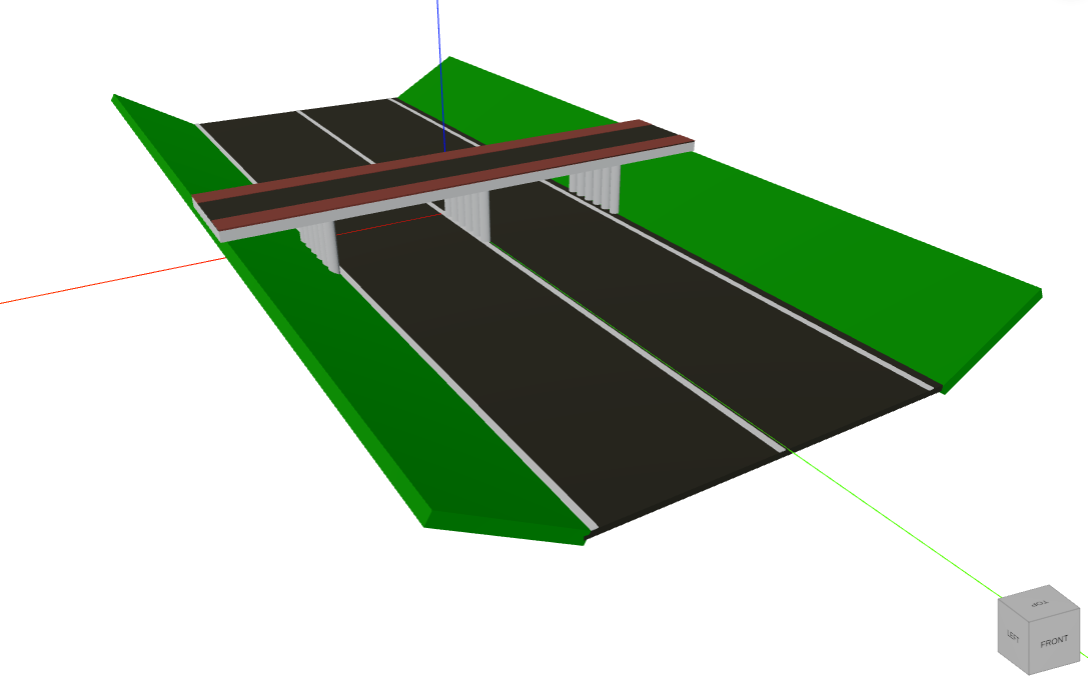# Show a 3D model

This guide explains how to visualize 3D models. There are multiple ways to achieve this:

1. From geometry elements from the `viktor.geometry` module
2. From a glTF/GLB file (>= v12.12.0)
3. From a 3DM file (>= v14.4.0)
4. From an IFC file (>= v14.6.0)

Depending on the method above, either a `GeometryView` or an `IFCView` needs to be used. Both views can be combined with data using a `GeometryAndDataView` and `IFCAndDataView`.## GeometryView​

### Geometry elements​

New in v13.5.0

`Arc`, `Line`, and `Polyline` can be visualized in the `GeometryView`

A `GeometryResult` can be constructed using geometry elements (`TransformableObject`) from the `viktor.geometry` module. The created 3D visualization uses a right-handed axis system with Z pointing upwards by default (see view settings to overwrite this).

#### Groups​

A visualization group (`Group`) can be used to combine multiple visualization elements. A `Group` can contain two types of objects: `Group`, `TransformableObject`. This enables a hierarchical construction of visualization elements.

Example:

``from viktor import Colorfrom viktor.geometry import Group, LinearPattern, SquareBeam, Materialfrom viktor.views import GeometryResult, GeometryViewclass Controller(ViktorController):    ...    @GeometryView("Geometry", duration_guess=1)    def get_geometry_view(self, params, **kwargs):        # Define Materials        glass = Material("Glass", color=Color(150, 150, 255))        facade = Material("Facade", color=Color.white())        # Create one floor        width = 30        length = 30        number_of_floors = 20        floor_glass = SquareBeam(width, length, 2, material=glass)        floor_facade = SquareBeam(width + 1, length + 1, 1, material=facade)        floor_facade.translate([0, 0, 1.5])            # Pattern (duplicate) the floor to create a building        floor = Group([floor_glass, floor_facade])        building = LinearPattern(floor, direction=[0, 0, 1], number_of_elements=number_of_floors, spacing=3)            return GeometryResult(building)``

#### Specifying color​

Every `TransformableObject` has an assigned default material. This material can be overwritten with your own, specifying, for example, the color:

``from viktor.geometry import Materialfrom viktor import Color...glass = SquareBeam(..., material=Material("Glass", color=Color(150, 150, 255)))# orglass = SquareBeam(...)glass.material = Material("Glass", color=Color(150, 150, 255))``

#### Transformations​

Transformation methods can be called on a `TransformableObject`, to position and orientate the object. The `Vector` object can come in handy if your visualization requires vector transformations. The following methods are available on all geometric objects (through inheritance of `TransformableObject`):

For each type of transformation an example is provided below. Chaining of transformations is possible, however be careful with the specified order.

##### translate​
``from viktor.geometry import Point, Spheresphere = Sphere(Point(0, 0, 0), radius=10)# sphere is now centered around (0, 0, 0)sphere.translate([10, 0, 0])# sphere is now centered around (10, 0, 0)``
##### rotate​
``import mathfrom viktor.geometry import Line, Point, RectangularExtrusionbox = RectangularExtrusion(1, 1, line=Line(Point(0, 0, -0.5), Point(0, 0, 0.5)))# box is now centered around (0, 0, 0)box.rotate(math.pi / 4, direction=[0, 0, 1])# box is now rotated 45 degrees around the z-axis according to the right-hand-rule``
##### mirror​
``from viktor.geometry import Point, Spheresphere = Sphere(Point(0, 0, 1), radius=10)# sphere is now centered around (0, 0, 1)sphere.mirror(Point(0, 0, 0), [0, 0, 1])# sphere is a now mirrored in the x-y plane (normal vector in z-direction), centered around (0, 0, -1)``
##### scale​
``from viktor.geometry import Point, Spheresphere = Sphere(Point(0, 0, 1), radius=10)# sphere is now centered around (0, 0, 1)sphere.scale([2, 2, 1])# sphere is stretched in x- and y-direction, centered around (0, 0, 1)``

### glTF/GLB file​

New in v12.12.0

A `GeometryResult` can also be constructed from a glTF/GLB (v2.0) file. This can be an existing local model:

``from viktor.views import GeometryView, GeometryResultclass Controller(ViktorController):    ...    @GeometryView('Geometry view', duration_guess=1)    def get_geometry_view(self, params, **kwargs):        geometry = File.from_path(Path(__file__).parent / "my_model.gltf")  # or .glb        return GeometryResult(geometry)``

Or one available on the internet:

``geometry = File.from_url("https://github.com/KhronosGroup/glTF-Sample-Models/raw/master/2.0/CesiumMilkTruck/glTF-Binary/CesiumMilkTruck.glb")return GeometryResult(geometry)``

Or can be generated by means of trimesh (or any other Python geometry package that allows for exporting to glTF/GLB):

``import trimesh...sphere = trimesh.creation.uv_sphere(10)scene = trimesh.Scene(geometry={'sphere': sphere})geometry = File()  # create a writable filewith geometry.open_binary() as w:    w.write(trimesh.exchange.gltf.export_glb(scene))return GeometryResult(geometry)``
note

glTF/GLB models are defined with the Y-axis up. In VIKTOR the default up-axis is the Z-axis, resulting in the model to be shown on its side. In order to overwrite this, you can set the `up_axis` manually in the view settings:

``@GeometryView("My glTF View", up_axis='Y')...``

### Rhinoceros 3DM file​

New in v14.4.0

Similar to a glTF/GLB file, a 3DM file can be visualized by specifying the `geometry_type` argument (default: "gltf"):

``geometry = File.from_url("https://github.com/mrdoob/three.js/raw/master/examples/models/3dm/Rhino_Logo.3dm")return GeometryResult(geometry, geometry_type="3dm")``

### Labels​

Regardless of how the geometry is defined (geometry elements, glTF/GLB, or 3DM), additional labels can be added to the visualization:

``from viktor.views import Labelfrom viktor.geometry import Point...labels = [    Label(Point(0, 0, 0), "Origin"),    Label(Point(1, 0, 0), "x=1")]return GeometryResult(solar_system, labels=labels)``

### View settings​

The following settings can be applied to a `GeometryView`:

• view_mode: str value to switch between '3D' (default) and '2D'. The created 2D visualization shows the xy-plane.
• default_shadow: bool value to define whether shadow is turned off (default) or on when entering the editor. User can still overrule.
• up_axis (>= v12.12.0): str value ('Y' or 'Z') to define the upwards pointing axis (view_mode = '3D' only, default: 'Z').

Example:

``@GeometryView("Top view", duration_guess=1, view_mode='2D', default_shadow=False)...``

## IFCView​

New in v14.6.0

An IFC file can be visualized by using the `IFCView`. This can be an existing local model:

``from viktor.views import IFCView, IFCResultclass Controller(ViktorController):    ...    @IFCView('IFC view', duration_guess=1)    def get_ifc_view(self, params, **kwargs):        ifc = File.from_path(Path(__file__).parent / 'sample.ifc')        return IFCResult(ifc)``

Or one available on the internet:

``ifc = File.from_url("https://github.com/IFCjs/test-ifc-files/raw/main/Others/haus.ifc")return IFCResult(ifc)``

## Testing​

New in v13.3.0

`mock_View` decorator for easier testing of view methods

Methods decorated with `@GeometryView`, `@GeometryAndDataView`, `@IFCView`, or `@IFCAndDataView` need to be mocked within the context of (automated) testing.

``import unittestfrom viktor.testing import mock_Viewfrom app.my_entity_type.controller import MyEntityTypeControllerclass TestMyEntityTypeController(unittest.TestCase):    @mock_View(MyEntityTypeController)    def test_geometry_view(self):        params = ...        result = MyEntityTypeController().geometry_view(params=params)        self.assertEqual(result.geometry, ...)        self.assertEqual(result.labels, ...)``